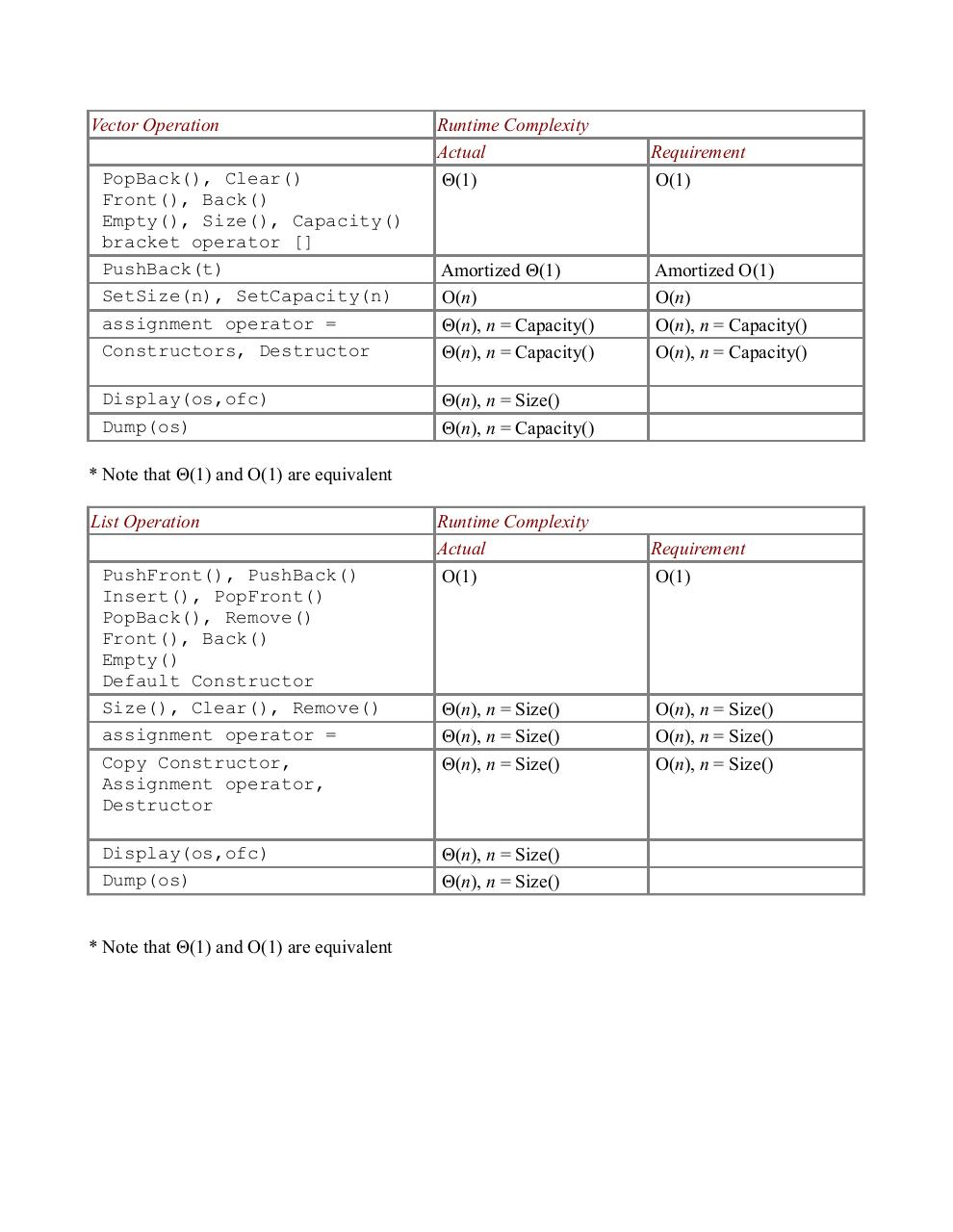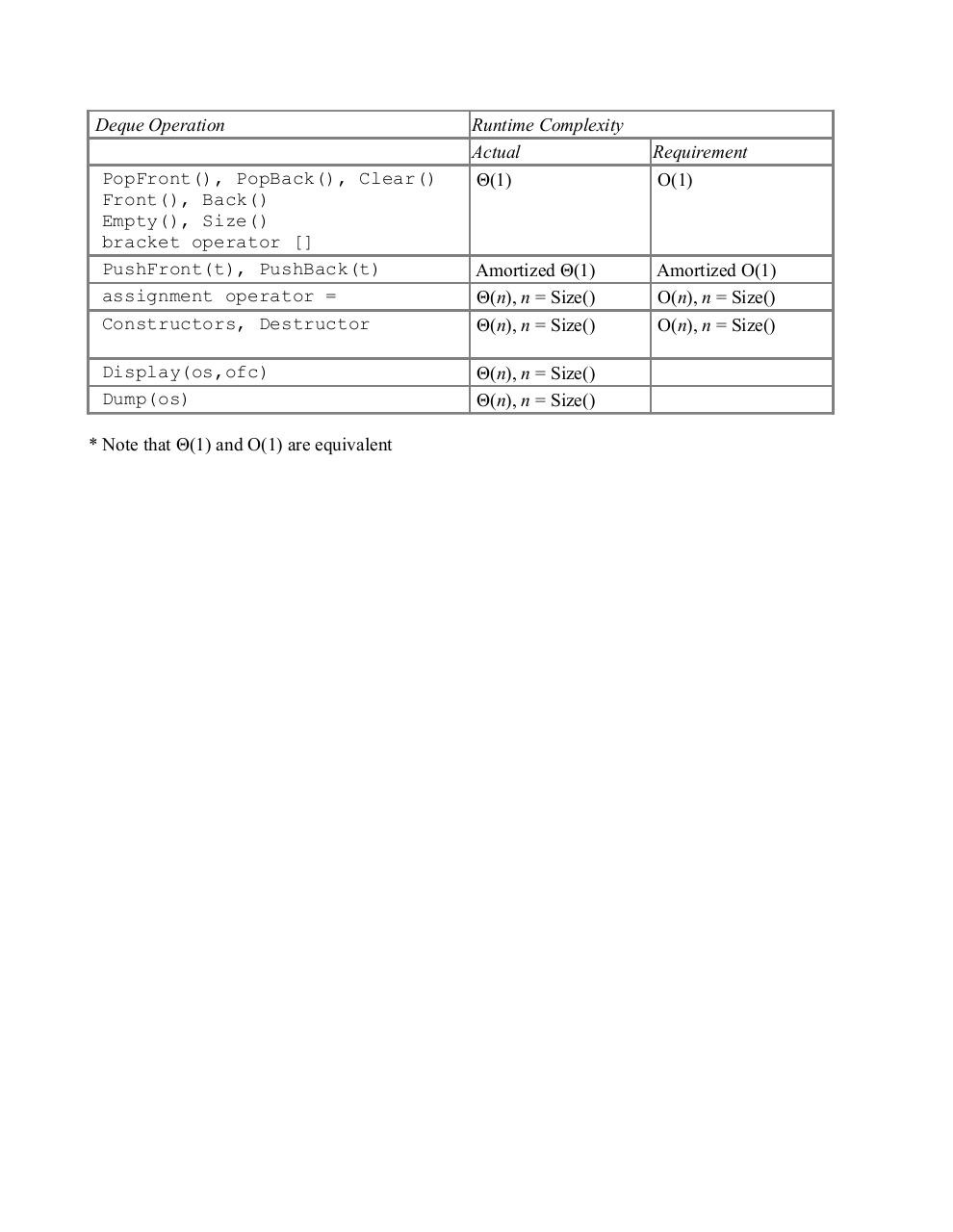# complexity .pdf

### File information

Original filename: complexity.pdf

This PDF 1.4 document has been generated by Writer / OpenOffice.org 3.4.1, and has been sent on pdf-archive.com on 26/06/2015 at 02:05, from IP address 98.183.x.x. The current document download page has been viewed 344 times.
File size: 62 KB (2 pages).
Privacy: public file

## Download original PDF file

complexity.pdf (PDF, 62 KB)

### Document preview

Vector Operation
PopBack(), Clear()
Front(), Back()
Empty(), Size(), Capacity()
bracket operator []
PushBack(t)
SetSize(n), SetCapacity(n)
assignment operator =
Constructors, Destructor
Display(os,ofc)
Dump(os)

Runtime Complexity
Actual
Θ(1)

Requirement
O(1)

Amortized Θ(1)
O(n)
Θ(n), n = Capacity()
Θ(n), n = Capacity()

Amortized O(1)
O(n)
O(n), n = Capacity()
O(n), n = Capacity()

Θ(n), n = Size()
Θ(n), n = Capacity()

* Note that Θ(1) and O(1) are equivalent
List Operation
PushFront(), PushBack()
Insert(), PopFront()
PopBack(), Remove()
Front(), Back()
Empty()
Default Constructor
Size(), Clear(), Remove()
assignment operator =
Copy Constructor,
Assignment operator,
Destructor
Display(os,ofc)
Dump(os)
* Note that Θ(1) and O(1) are equivalent

Runtime Complexity
Actual
O(1)

Requirement
O(1)

Θ(n), n = Size()
Θ(n), n = Size()
Θ(n), n = Size()

O(n), n = Size()
O(n), n = Size()
O(n), n = Size()

Θ(n), n = Size()
Θ(n), n = Size()

Deque Operation
PopFront(), PopBack(), Clear()
Front(), Back()
Empty(), Size()
bracket operator []
PushFront(t), PushBack(t)
assignment operator =
Constructors, Destructor
Display(os,ofc)
Dump(os)
* Note that Θ(1) and O(1) are equivalent

Runtime Complexity
Actual
Θ(1)

Requirement
O(1)

Amortized Θ(1)
Θ(n), n = Size()
Θ(n), n = Size()

Amortized O(1)
O(n), n = Size()
O(n), n = Size()

Θ(n), n = Size()
Θ(n), n = Size()### Link to this page

#### Permanent link

Use the permanent link to the download page to share your document on Facebook, Twitter, LinkedIn, or directly with a contact by e-Mail, Messenger, Whatsapp, Line..

#### Short link

Use the short link to share your document on Twitter or by text message (SMS)

#### HTML Code

Copy the following HTML code to share your document on a Website or Blog

#### QR Code### Related keywords